# PASS Summit And Other Speaking And Training Dates

This is just a quick post to advertise some upcoming speaking and training dates…

PASS Summit

I’ll be at the PASS Summit in Seattle next week, and if you see me around be sure to say hello! I’ll be presenting a deep dive session on MDX scoped assignments at 9:45am on Friday November 7th, and I’ll be signing copies of my new Power Query book on the Apress stand in the exhibition hall at 1pm on Wednesday November 5th.

I will also be live-tweeting (I’m @technitrain on Twitter if you aren’t following me already) and blogging throughout the keynotes each day too. I suspect there will be some big announcements this year – Jen Underwood dropped some hints, and a list of interesting sessions to attend, on her blog last week.

Future Decoded

On Wednesday November 12th I’ll be speaking about Power BI at Microsoft’s Future Decoded event in London, alongside the likes of Jen Stirrup, James Rowland-Jones and assorted celebrities like Professor Brian Cox, Sir Nigel Shadbolt and David Braben (the guy who co-wrote Elite!)

Training in London

There are a whole bunch of new courses up on the Technitrain site, taught by me and various other SQL Server experts (such as James Rowland-Jones, Jamie Thomson, Klaus Aschenbrenner, Andy Leonard and Allan Hirt) from around the world, and covering a wide variety of SQL Server and Microsoft BI topics. There are still places available on my Power Query course on Thursday November 13th if you’re interested in attending.

Training in Aarhus, Denmark

In December I’ll be teaching two one-day courses with my friends at Orange Man in Aarhus in Denmark: an introduction to Power BI on December 3rd and my Power Query course again on December 4th.

# BI Survey 14 Results

Once again, the nice people at BARC have sent me a copy of the results of the latest BI Survey and allowed me to blog about some of their findings (obviously if you want to read them all, buy the survey!). Here are a couple of things that caught my eye:

• When respondents were asked about which BI products they evaluated, Qlik came top of the list with 36% evaluating it, followed by all of the Microsoft products (Excel/Power BI at 35%, SSAS at 28% and SSRS at 26%). However when it came to picking products Excel/Power BI came top at 25%, followed by ‘other’ products, then SSAS at 21% and SSRS at 17% with Qlik at 16%. I wonder what parts of the Power BI stack the Excel/Power BI users were actually using exactly though? I suppose the point about it is that users can take whatever parts of it they want to complement what they do in Excel. These numbers are very encouraging in any case.
• Looking at reported usage problems for MS products some familiar issues came up: 25% of Excel/Power BI users complained that the product couldn’t handle the data volumes they wanted and 16% complained of security limitations – both scores were the worst across all products. Partly this can be explained by the desktop-bound nature of the product, but I wonder whether the limitations of 32 bit Excel are behind the data volume problems? Also, 18% of SSRS users complained of missing key features, which again was the worst score for this category across all products. I hope MS plans to show SSRS some more love in the future after several years of neglect. Other products have other weaknesses of course – 26% of Tableau users had administrative problems, 53% of SAP BW users had problems with slow query performance and 21% of TM1 users had issues with poor data governance. Nothing is perfect.
• Respondents were asked about cloud BI adoption. For those using Excel/Power BI, 15% were in the cloud now (the third best score across all products) which I assume means they are using Power BI for Office 365; a further 15% were planning to go to the cloud in the next 12 months; a further 19% were planning to go in the long term; and 51% had no plans. Presumably this last group of users would like to see more of the Power BI for Office 365 functionality implemented within SharePoint on premises.

# Sentiment Analysis In Excel With Azure Machine Learning And Power Query

You may have seen Joseph Sirosh’s blog post last week about the ability to publish Azure Machine Learning models to the Azure Marketplace, and that MS have published a number of APIs there already. There’s a new Excel add-in that can be used with these APIs but I noticed that at least one of them, the Sentiment Analysis API, can be used direct from Power Query too.

To do this, the first thing you need to do is to go to the Azure Marketplace, sign in with your Microsoft account, and subscribe to the Lexicon Based Sentiment Analysis API. The docs say you get 25000 transactions free per month although there doesn’t appear to be a way to pay for more; that said the number of transactions remaining shown on my account kept resetting, so maybe there is no limit. The API itself is straightforward: pass it a sentence to evaluate and it will return a score between –1 and 1, where 1 represents a positive sentiment and –1 is a negative sentiment. For example, the sentence “I had a good day” returns the value 1:You can now go to Power Query and click From Azure Marketplace (you will need to enter your credentials at this point if this is the first time you’ve used the Azure Marketplace from Power Query):…and then, when the Navigator pane appears, double-click on Score:The API is exposed as a function in Power Query (I guess because it’s an OData service operation, but I’m not sure) and double-clicking on Score invokes the function. You can enter a sentence here and the Query Editor will open to show the score: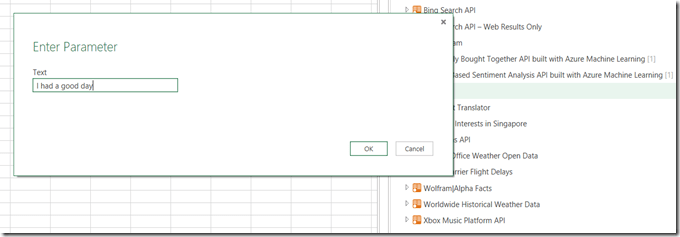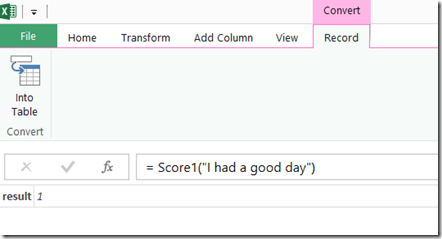However, to do anything useful you don’t want to invoke the function just yet – you want a query that returns the function. To do this you need to edit the query. Go to the Advanced Editor and you’ll see the M code for the query will be something like this:

```let
Source = Marketplace.Subscriptions(),
#&quot;https://api.datamarket.azure.com/data.ashx/aml_labs/
lexicon_based_sentiment_analysis/v1/&quot; =
Source{
[ServiceUrl=&quot;https://api.datamarket.azure.com/data.ashx/aml_labs/
lexicon_based_sentiment_analysis/v1/&quot;]
}[Feeds],
Score1 =
#&quot;https://api.datamarket.azure.com/data.ashx/aml_labs/
lexicon_based_sentiment_analysis/v1/&quot;
{[Name=&quot;Score&quot;]}[Data],
#&quot;Invoked FunctionScore1&quot; = Score1(&quot;I had a good day&quot;)
in
#&quot;Invoked FunctionScore1&quot;

```

You need to remove the last line (called #”Invoked FunctionScore1”) which invokes the function, leaving:

```let
Source = Marketplace.Subscriptions(),
#&quot;https://api.datamarket.azure.com/data.ashx/aml_labs/
lexicon_based_sentiment_analysis/v1/&quot; =
Source{[ServiceUrl=&quot;https://api.datamarket.azure.com/data.ashx/aml_labs/
lexicon_based_sentiment_analysis/v1/&quot;]}[Feeds],
Score1 = #&quot;https://api.datamarket.azure.com/data.ashx/aml_labs/
lexicon_based_sentiment_analysis/v1/&quot;
{[Name=&quot;Score&quot;]}[Data]
in
Score1

```

You can now click the Close and Load button to close the Query Editor window; you now have a function called Score that you can call in other queries. For example, take the following Excel table (called Sentences):Click on the From Table button to load this table into Power Query, then in the Query Editor click the Add Custom Column button and add a new custom column called Sentiment Score with the expression

Score([Sentence])You’ll then be prompted to set a data privacy level for the data you’re using, because calling this function involves sending data from your worksheet up to the API where someone could potentially see it.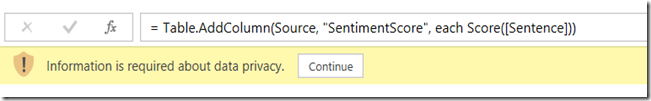Click the Continue button and set the privacy level for the workbook to Public, so that this data can be sent to the API: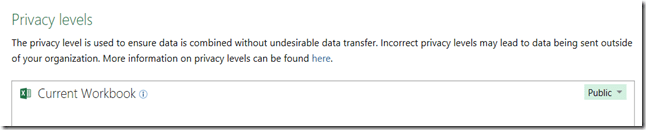Click Save and you’ll see the sentiment score has been added as a column containing a Record value. Click on the Expand icon in the SentimentScore column then OK: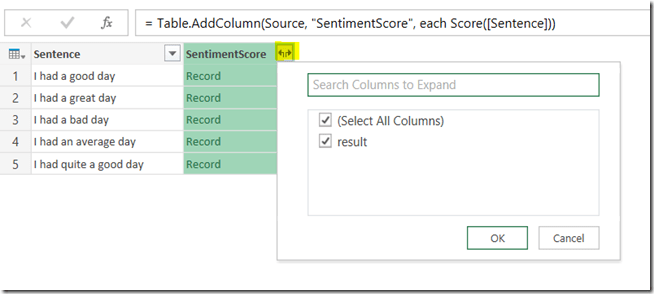And at last you’ll see the scores themselves:Here’s the code:

```let
Source = Excel.CurrentWorkbook(){[Name=&quot;Sentences&quot;]}[Content],
#&quot;Expand SentimentScore&quot; =
{&quot;result&quot;}, {&quot;SentimentScore.result&quot;})
in
#&quot;Expand SentimentScore&quot;

```

Of course, I couldn’t resist repeating this exercise with all of my Facebook status updates – which, of course, can be accessed from Power Query very easily. Here’s my monthly average sentiment score from June 2009 to now:As you can see, I was in a particularly good mood this August – probably because I was on holiday for almost the whole month.

# MDX Solve Order, SCOPE_ISOLATION and the Aggregate() function

Solve order in MDX is a mess. Back in the good old days of Analysis Services 2000 it was a difficult concept but at least comprehensible; unfortunately when Analysis Services 2005 was released a well-intentioned attempt at making it easier to work with in fact ended up making things much, much worse. In this post I’m going to summarise everything I know about solve order in MDX to try to make this complicated topic a little bit easier to understand.

If you’re an experienced MDXer, at this point you’ll probably lose interest because you think you know everything there is to know about solve order already. Up until two weeks ago that’s what I though too, so even if you know everything I say in the first half of this post keep reading – there’s some new stuff at the end I’ve only just found out about.

Let’s start with a super-simple cube built from a single table, with two measures (Sales Amount and Cost Amount) and a Product dimension containing a single attribute hierarchy with two members (Apples and Oranges). Everything is built from the following table:Solve Order and calculated members in the WITH clause

To understand what solve order is and how it can be manipulated, let’s start off looking at an example that uses only calculated members in the WITH clause of a query. Consider the following:

`WITH`

` `

`MEMBER [Measures].[Cost %] AS`

`DIVIDE([Measures].[Cost Amount],[Measures].[Sales Amount]),`

`FORMAT_STRING='0.0%'`

` `

`MEMBER [Product].[Product].[Total Fruit] AS`

`SUM({[Product].[Product].&[Apples],`

`[Product].[Product].&[Oranges]})`

` `

`SELECT`

`{[Measures].[Sales Amount],`

`[Measures].[Cost Amount],`

`MEASURES.[Cost %]}`

`ON COLUMNS,`

`{[Product].[Product].&[Apples],`

`[Product].[Product].&[Oranges],`

`[Product].[Product].[Total Fruit]}`

`ON ROWS`

`FROM SALES`

There are two calculated members here:

• Cost % divides Cost Amount by Sales Amount to find the percentage that costs make up of the sales amount
• Total Fruit sums up the values for Apples and Oranges

The output of the query is as follows:Solve order controls the order that MDX calculations are evaluated when two or more of them overlap in the same cell. In this case Cost % and Total Fruit are both evaluated in the bottom right-hand cell; Total Fruit is calculated first, giving the values of 30 for Sales Amount and 21 for Cost Amount, and Cost % is calculated after that. The bottom right-hand cell is the only cell where these two calculations overlap and the only cell where solve order is relevant in this query.

In this case, 70% is the value you would expect to get. You, however, can control solve order for calculations in the WITH clause by setting the SOLVE_ORDER property for each calculated member, like so:

`WITH`

` `

`MEMBER [Measures].[Cost %] AS`

`DIVIDE([Measures].[Cost Amount],[Measures].[Sales Amount]),`

`FORMAT_STRING='0.0%',`

`SOLVE_ORDER=1`

` `

`MEMBER [Product].[Product].[Total Fruit] AS`

`SUM({[Product].[Product].&[Apples],`

`[Product].[Product].&[Oranges]}),`

`SOLVE_ORDER=2`

` `

`SELECT`

`{[Measures].[Sales Amount],`

`[Measures].[Cost Amount],`

`MEASURES.[Cost %]}`

`ON COLUMNS,`

`{[Product].[Product].&[Apples],`

`[Product].[Product].&[Oranges],`

`[Product].[Product].[Total Fruit]}`

`ON ROWS`

`FROM SALES`Now the value in the bottom right-hand corner is 135% instead of 70%: Cost % is calculated first, then Total Fruit second so 60%+75%=135%. The SOLVE_ORDER property of a calculated member is an integer value, and the lower the SOLVE_ORDER value the earlier the calculation will be evaluated, so with Cost % having a solve order of 1 and Total Fruit having a solve order of 2, this forces Cost % to be calculated first now even though in this case it gives what is clearly an ‘incorrect’ result.

Solve Order and calculated members defined on the cube

Things now get a bit more complicated. There’s a different way of controlling solve order if your calculations are defined on the cube itself: in this case, solve order is determined by the order that the calculations appear on the Calculations tab. So if the calculations tab of the Cube Editor contains the calculations in this order:

`CREATE MEMBER CURRENTCUBE.[Measures].[Cost %] AS`

`DIVIDE([Measures].[Cost Amount],[Measures].[Sales Amount]),`

`FORMAT_STRING='0.0%';`

` `

`CREATE MEMBER CURRENTCUBE.[Product].[Product].[Total Fruit] AS`

`SUM({[Product].[Product].&[Apples],`

`[Product].[Product].&[Oranges]});`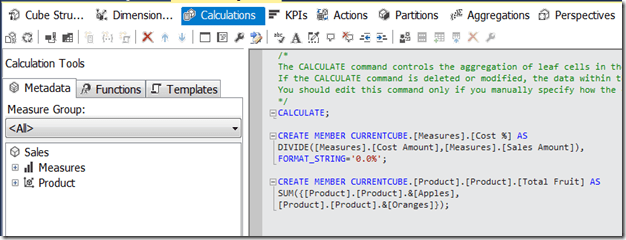…and you run the following query:

`SELECT`

`{[Measures].[Sales Amount],`

`[Measures].[Cost Amount],`

`MEASURES.[Cost %]}`

`ON COLUMNS,`

`{[Product].[Product].&[Apples],`

`[Product].[Product].&[Oranges],`

`[Product].[Product].[Total Fruit]}`

`ON ROWS`

`FROM SALES`

You get the incorrect result again: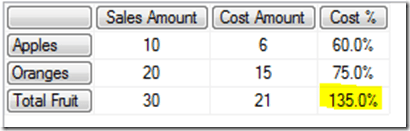…but if you change the order of the calculations so that Total Fruit comes first:…and rerun the same query, you get the correct results: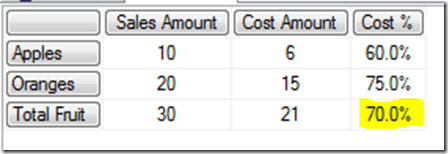The SOLVE_ORDER property can also be used with calculations defined on the cube to override the effect of the order of calculations. So defining the following calculations on the cube:

`CREATE MEMBER CURRENTCUBE.MEASURES.[Cost %] AS`

`DIVIDE([Measures].[Cost Amount], [Measures].[Sales Amount]),`

`FORMAT_STRING='PERCENT', SOLVE_ORDER=2;`

` `

`CREATE MEMBER CURRENTCUBE.[Product].[Product].[Total Fruit] AS`

`SUM({[Product].[Product].&[Apples],`

`[Product].[Product].&[Oranges]}), SOLVE_ORDER=1;`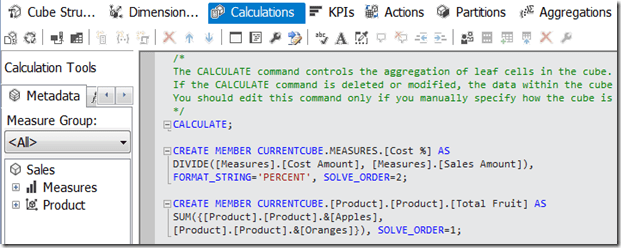…means that, even though Total Fruit comes after Cost % on the Calculations tab, because it has a lower solve order set using the SOLVE_ORDER property it is evaluated before Cost % and the query still returns the correct value: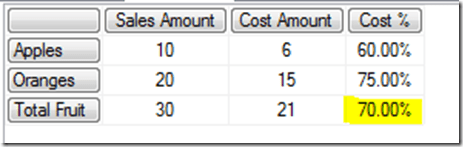Solve order and calculations defined in the WITH clause and on the cube

What happens if some calculations are defined on the cube, and some are defined in the WITH clause of a query? By default, calculations defined on the cube always have a lower solve order than calculations defined in the WITH clause of a query; the SOLVE_ORDER property has no effect here. So if Total Fruit is defined in the WITH clause and Cost % on the cube, you get the incorrect result:`WITH`

` `

`MEMBER [Product].[Product].[Total Fruit] AS`

`SUM({[Product].[Product].&[Apples],`

`[Product].[Product].&[Oranges]})`

` `

`SELECT`

`{[Measures].[Sales Amount],`

`[Measures].[Cost Amount],`

`MEASURES.[Cost %]}`

`ON COLUMNS,`

`{[Product].[Product].&[Apples],`

`[Product].[Product].&[Oranges],`

`[Product].[Product].[Total Fruit]}`

`ON ROWS`

`FROM SALES`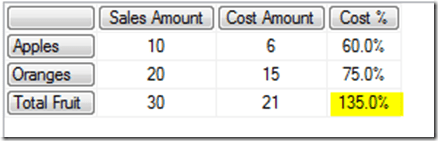Of course, if Total Fruit is defined on the cube and Cost % is defined in the WITH clause you will get the correct answer. However, usually measures like Cost % are defined on the cube and it’s calculations like Total Fruit, which define custom groupings, that are defined on an ad hoc basis in the WITH clause. This is a problem.

The SCOPE_ISOLATION property

This default behaviour of calculations defined on the cube always having a lower solve order than calculations in the WITH clause can be overridden using the SCOPE_ISOLATION property. Setting SCOPE_ISOLATION=CUBE for a calculated member defined in the WITH clause will give that calculated member a lower solve order than any calculations defined on the cube. So, with Cost % still defined on the cube the following query now gives the correct results:

`WITH`

` `

`MEMBER [Product].[Product].[Total Fruit] AS`

`SUM({[Product].[Product].&[Apples],`

`[Product].[Product].&[Oranges]}),`

`SCOPE_ISOLATION=CUBE`

` `

`SELECT`

`{[Measures].[Sales Amount],`

`[Measures].[Cost Amount],`

`MEASURES.[Cost %]}`

`ON COLUMNS,`

`{[Product].[Product].&[Apples],`

`[Product].[Product].&[Oranges],`

`[Product].[Product].[Total Fruit]}`

`ON ROWS`

`FROM SALES`The Aggregate() function

Using the MDX Aggregate() function (and in fact also the VisualTotals() function – but you probably won’t ever want to use it) inside a calculation has a similar effect to the SCOPE_ISOLATION property in that it forces a calculation to be evaluated at a lower solve order than anything else. Therefore, in the previous example, instead of using the SCOPE_ISOLATION property you can change the calculation to use the Aggregate() function instead of Sum() and get the correct results:

`WITH`

` `

`MEMBER [Product].[Product].[Total Fruit] AS`

`AGGREGATE({[Product].[Product].&[Apples],`

`[Product].[Product].&[Oranges]})`

` `

`SELECT`

`{[Measures].[Sales Amount],`

`[Measures].[Cost Amount],`

`MEASURES.[Cost %]}`

`ON COLUMNS,`

`{[Product].[Product].&[Apples],`

`[Product].[Product].&[Oranges],`

`[Product].[Product].[Total Fruit]}`

`ON ROWS`

`FROM SALES`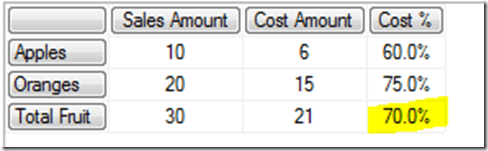The general rule is, therefore, whenever you are creating custom-grouping type calculated members like Total Fruit in the WITH clause of a query, to use the Aggregate() function rather than Sum(). The fact that Aggregate() takes into account the AggregateFunction property of each measure on the cube (so that distinct count, min and max measures are dealt with correctly) is another good reason to use it.

Using the Aggregate() function in calculations defined on the cube has the same effect. Even when the Total Fruit calculated member is defined after Cost % on the Calculations tab, as here:…so long as Total Fruit uses the Aggregate() function, running the test query gives the correct result:

`SELECT`

`{[Measures].[Sales Amount],`

`[Measures].[Cost Amount],`

`MEASURES.[Cost %]}`

`ON COLUMNS,`

`{[Product].[Product].&[Apples],`

`[Product].[Product].&[Oranges],`

`[Product].[Product].[Total Fruit]}`

`ON ROWS`

`FROM SALES`

There are some very interesting details about the way Aggregate() changes solve order though.

First of all, using the Aggregate() function in a calculated member doesn’t change the solve order of the whole calculation, just the part of the calculation that uses the Aggregate() function. With the following calculations defined on the cube:

`CREATE MEMBER CURRENTCUBE.[Measures].[Cost %] AS`

`DIVIDE([Measures].[Cost Amount],[Measures].[Sales Amount]),`

`FORMAT_STRING='0.0%';`

` `

`CREATE MEMBER CURRENTCUBE.[Product].[Product].[One Aggregate] AS`

`AGGREGATE({[Product].[Product].&[Apples],`

`[Product].[Product].&[Oranges]});`

` `

`CREATE MEMBER CURRENTCUBE.[Product].[Product].[One Sum] AS`

`SUM({[Product].[Product].&[Apples],`

`[Product].[Product].&[Oranges]});`

` `

`CREATE MEMBER CURRENTCUBE.[Product].[Product].[Two Aggregates] AS`

`AGGREGATE({[Product].[Product].&[Apples],`

`[Product].[Product].&[Oranges]})`

`+`

`AGGREGATE({[Product].[Product].&[Apples],`

`[Product].[Product].&[Oranges]});`

` `

`CREATE MEMBER CURRENTCUBE.[Product].[Product].[Two Sums] AS`

`SUM({[Product].[Product].&[Apples],`

`[Product].[Product].&[Oranges]})`

`+`

`SUM({[Product].[Product].&[Apples],`

`[Product].[Product].&[Oranges]});`

` `

`CREATE MEMBER CURRENTCUBE.[Product].[Product].[One Aggregate One Sum] AS`

`AGGREGATE({[Product].[Product].&[Apples],`

`[Product].[Product].&[Oranges]})`

`+`

`SUM({[Product].[Product].&[Apples],`

`[Product].[Product].&[Oranges]});`

…running the following query:

`SELECT`

`{[Measures].[Sales Amount],`

`[Measures].[Cost Amount],`

`MEASURES.[Cost %]}`

`ON COLUMNS,`

`{[Product].[Product].&[Apples],`

`[Product].[Product].&[Oranges],`

`[Product].[Product].[One Aggregate],`

`[Product].[Product].[One Sum],`

`[Product].[Product].[Two Aggregates],`

`[Product].[Product].[Two Sums],`

`[Product].[Product].[One Aggregate One Sum]}`

`ON ROWS`

`FROM SALES`

…gives these results:The value returned for the calculation [One Aggregate One Sum], which contains an Aggregate() and a Sum(), shows that the value returned by the Aggregate() is evaluated at a different solve order than the value returned by Sum(), even if they are inside the same calculated member.

Furthermore, in some very obscure cases the contents of the set passed to the Aggregate() function determine whether its special solve order behaviour happens or not. I don’t know for sure what all those cases are but I have seen this happen with time utility (aka date tool aka shell) dimensions. Here’s an example.

The demo cube I’ve been using in this post has been changed to add a new dimension, called Data Type, which has just one hierarchy with one member on it called Actuals; Data Type is a fairly standard time utility dimension. The Cost % calculation has also been changed so that it’s now a calculated member on the Data Type dimension, although it is still defined on the cube. Here’s its new definition:

`CREATE MEMBER CURRENTCUBE.[Data Type].[Data Type].[Cost %] AS`

`DIVIDE(`

`([Measures].[Cost Amount],[Data Type].[Data Type].&[Actuals]),`

`([Measures].[Sales Amount],[Data Type].[Data Type].&[Actuals])),`

`FORMAT_STRING='0.0%';`

Now if I run the following query:

`WITH`

` `

`MEMBER [Product].[Product].[Simple Set] AS`

`AGGREGATE({[Product].[Product].&[Apples],`

`[Product].[Product].&[Oranges]})`

` `

`MEMBER [Product].[Product].[Nextmember Function Used] AS`

`AGGREGATE({[Product].[Product].&[Apples],`

`[Product].[Product].&[Apples].NEXTMEMBER})`

` `

`MEMBER [Product].[Product].[Descendants Function Used] AS`

`AGGREGATE(DESCENDANTS({[Product].[Product].&[Apples],`

`[Product].[Product].&[Oranges]}))`

` `

`MEMBER [Product].[Product].[Descendants Function Used Twice] AS`

`AGGREGATE({`

`DESCENDANTS([Product].[Product].&[Apples]),`

`DESCENDANTS([Product].[Product].&[Oranges])`

`})`

` `

`MEMBER [Product].[Product].[Descendants Function Used Twice With Union] AS`

`AGGREGATE(`

`UNION(`

`DESCENDANTS([Product].[Product].&[Apples]),`

`DESCENDANTS([Product].[Product].&[Oranges])`

`))`

` `

`SELECT`

`{[Measures].[Sales Amount]}`

`*`

`[Data Type].[Data Type].ALLMEMBERS`

`ON COLUMNS,`

`{[Product].[Product].&[Apples],`

`[Product].[Product].&[Oranges],`

`[Product].[Product].[Simple Set],`

`[Product].[Product].[Nextmember Function Used],`

`[Product].[Product].[Descendants Function Used],`

`[Product].[Product].[Descendants Function Used Twice],`

`[Product].[Product].[Descendants Function Used Twice With Union]}`

`ON ROWS`

`FROM [Sales With Data Type]`

I get these results:Note that for some of the calculations, the Aggregate() function results in a lower solve order in the way we’ve already seen, but not for all of them. Using the NextMember() function, or having two Descendants() functions without wrapping them in a Union() function, seems to stop SSAS assigning the calculation a lower solve order. Ugh. Luckily, though, I have only been able to replicate this with calculated members from two non-measures dimensions; if Cost % is a calculated measure Aggregate() always gives the lower solve order. Apparently this is something that SSAS does on purpose to try to recognise ‘visual total’-like calculated members and make them work the way you want automatically. This is definitely something to beware of if you are using time utility dimensions and calculations on other dimensions though, as it may result in incorrect values being displayed or performance problems if you’re not careful.

[Thanks to Gabi Münster for showing me how Aggregate() works with different sets and Marius Dumitru for confirming that this is intended behaviour]

# Thoughts On Office Sway And BI

When I first saw the announcement about Office Sway last week, I thought – well, you can probably guess what I thought. Does it have any potential for BI? After all, the Sway team are clearly targeting business users (as well as hipster designers and schoolchildren): look at the Northwest Aquarium and Smith Fashion Expansion samples, and notice that they contain tables, charts and infographics. What’s more, data storytelling is currently a very hot concept and Sway is clearly all about telling stories. Wouldn’t it be cool if you could have interactive PivotTables, PivotCharts and Power View reports from your Power BI site embedded in a Sway? It would be a much more engaging way of presenting data than yet another PowerPoint deck.

I have no idea whether any integration between Sway and Power BI is actually planned (I have learned not to get my hopes up about this type of thing), but even if it isn’t maybe someone at Microsoft will read this post and think about the possibilities… And isn’t this kind of collaboration between different teams supposedly one of the advantages Microsoft has over its competitors in the BI space?

Office Sway introductory video

PS I want a pink octopus costume just like the one that girl in the video has

# Creating Histograms With Power Query

A few months ago someone at a conference asked me what the Power Query Table.Partition() function could be used for, and I had to admit I had no idea. However, when I thought about it, I realised one obvious use: for creating histograms! Now I know there are lots of other good ways to create histograms in Excel but here’s one more, and hopefully it will satisfy the curiosity of anyone else who is wondering about Table.Partition().

Let’s start with a table in Excel (called “Customers”) containing a list of names and ages: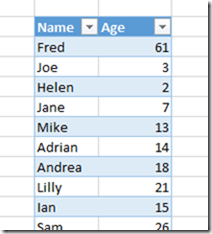Here’s the M code for the query to find the buckets:

`let`

`    //Get data from Customers table`

`    Source = Excel.CurrentWorkbook(){[Name="Customers"]}[Content],`

`    //Get a list of all the values in the Age column`

`    Ages = Table.Column(Source,"Age"),`

`    //Find the maximum age`

`    MaxAge = List.Max(Ages),`

`    //The number of buckets is the max age divided by ten, then rounded up to the nearest integer`

`    NumberOfBuckets = Number.RoundUp(MaxAge/10),`

`    //Hash function to determine which bucket each customer goes into`

`    BucketHashFunction = (age) => Number.RoundDown(age/10),`

`    //Use Table.Partition() to split the table into multiple buckets`

`    CreateBuckets = Table.Partition(Source, "Age", NumberOfBuckets, BucketHashFunction),`

`    //Turn the resulting list into a table`

`    #"Table from List" = Table.FromList(CreateBuckets, Splitter.SplitByNothing()`

`                           , null, null, ExtraValues.Error),`

`    //Add a zero-based index column`

`    #"Added Index" = Table.AddIndexColumn(#"Table from List", "Index", 0, 1),`

`    //Calculate the name of each bucket`

`    #"Added Custom" = Table.AddColumn(#"Added Index", "Bucket", `

`                        each Number.ToText([Index]*10) & " to " & Number.ToText(([Index]+1)*10)),`

`    //Find the number of rows in each bucket - ie the count of customers`

`    #"Added Custom1" = Table.AddColumn(#"Added Custom", "Count", each Table.RowCount([Column1])),`

`    //Remove unnecessary columns`

`    #"Removed Columns" = Table.RemoveColumns(#"Added Custom1",{"Column1", "Index"})`

`in`

`    #"Removed Columns"`

And here’s the output in Excel, with a bar chart:

How does this work?

• After loading the data from the Excel table in the Source step, the first problem is to determine how many buckets we’ll need. This is fairly straightforward: I use Table.Column() to get a list containing all of the values in the Age column, then use List.Max() to find the maximum age, then divide this number by ten and round up to the nearest integer.
• Now for Table.Partition(). The first thing to understand about this function is what it returns: it takes a table and returns a list of tables, so you start with one table and end up with multiple tables. Each row from the original table will end up in one of the output tables. A list object is something like an array.
• One of the parameters that the Table.Partition() function needs is a hash function that determines which bucket table each row from the original table goes into. The BucketHashFunction step serves this purpose here: it takes a value, divides it by ten and rounds the result down; for example pass in the age 88 and you get the value 8 back.
• The CreateBuckets step calls Table.Partition() with the four parameters it needs: the name of the table to partition, the column to partition by, the number of buckets to create and the hash function. For each row in the original table the age of each customer is passed to the hash function. The number that the hash function returns is the index of the table in the list that Table.Partition() returns. In the example above nine buckets are created, so Table.Partition() returns a list containing nine tables; for the age 8, the hash function returns 0 so the row is put in the table at index 0 in the list; for the age 88 the hash function returns 8, so the row is put in the table at index 8 in the list. The output of this step, the list of tables, looks like this:• The next thing to do is to convert the list itself to a table, then add a custom column to show the names for each bucket. This is achieved by adding a zero-based index column and then using that index value to generate the required text in the step #”Added Custom”.
• Next, find the number of customers in each bucket. Remember that at this point the query still includes a column (called “Column1”) that contains a value of type table, so all that is needed is to create another custom column that calls Table.RowCount() for each bucket table, as seen in the step #”Added Custom1”.
• Finally I remove the columns that aren’t needed for the output table.

I’m not convinced this is the most efficient solution for large data sets (I bet query folding stops very early on if you try this on a SQL Server data source) but it’s a good example of how Table.Partition() works. What other uses for it can you think of?

# The DirectSlice Property And Aggregations

A bit of an obscure one, this, but useful to know nonetheless…

You may or may not be aware of the DirectSlice property, which can be set on a many-to-many relationship in the Dimension Usage pane in Visual Studio: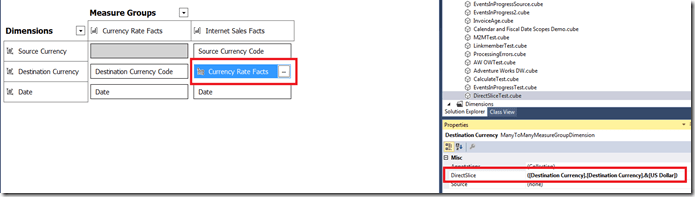One of the few good descriptions of it comes from this old white paper:

http://technet.microsoft.com/en-us/library/ms345139(v=sql.90).aspx

I quote:

When a measure expression is defined, it may be expensive to compute the result for the all member of the Many-to-Many dimension if the product needs to be computed across an enormous number of rows. And in examples like this, the value for the all member is the simple sum of the fact table. There is one more property on a many-to-many dimension that accommodates this, the Direct Slice. This is a tuple on the Many-to-Many dimension, where the server is instructed to not compute the measure expression except where the cube author guarantees that the aggregate value of the facts matches the result of this expression. For example, in a scenario using measure expression for currency conversion where the sales facts are stored in US Dollars, Currency.USD would be the DirectSlice in the Currency dimension.

Basically, what it does is let you tell SSAS to not evaluate the m2m relationship if a specific selection has been made and if doing so does not change the output of your query. Doing this could improve query performance if evaluating the m2m relationship is expensive – which it often is.

Here’s an example. The screenshot above shows a simplified example based on the Adventure Works cube containing two measure groups, Currency Rate Facts and Internet Sales Facts, and three dimensions, Source Currency, Destination Currency and Date. Destination Currency has a m2m relationship with the Internet Sales Facts measure group that is resolved through the Currency Rate Facts measure group; it also has IsAggregatable=False set and the US Dollar member is the default member.

Now, if you run a query to get data for a measure by year, like this:

`select`

`{[Measures].[Sales Amount]} on 0,`

`[Date].[Calendar].[Calendar Year].members on 1`

`from `

`[DirectSliceTest]`

…and look in Profiler, you’ll see that both the measure groups are being queried by the Storage Engine – as you would expect.What’s more, if you build an aggregation on the Internet Sales Facts measure group at the correct granularity, you’ll see this aggregation being used by the query:Incidentally, there’s a widely-repeated myth that you can’t use aggregations with m2m relationships or measure expressions – this is not true, although you do have to understand the granularity of data that the Storage Engine is querying and building your aggregation in the right place.

Now it’s time to set the DirectSlice property. In this case I set it to a tuple containing US Dollars, which is as I said earlier the default member on the Destination Currency dimension:

([Destination Currency].[Destination Currency].&[US Dollar])

Remember that by setting this property, I’m telling SSAS that it doesn’t need to evaluate the m2m relationship when this member is selected on Destination Currency because the results of any queries will be identical.

If you rerun the query then there are two things to notice in Profiler:First of all, the Currency Rates Facts measure group is no longer being queried, and so the m2m relationship is not being evaluated. This is good. Second, the aggregation seen above is no longer being used. In fact based on my research there’s no way to get an aggregation to be used when the DirectSlice property is set and the m2m relationship is being bypassed (and I’ve tried several variations, even building aggregations that include attributes from the Destination Currency dimension). This is not good. However as soon as you select something other than what is specified in the DirectSlice property, and the m2m relationship is being evaluated, aggregations can be used again.

In summary, then, using the DirectSlice property is a good idea if you have an expensive m2m relationship and you don’t expect your queries to use aggregations. However if you do need to use aggregations to improve query performance you probably should not set the DirectSlice property.

[Thanks to Akshai Mirchandani for helping with this]